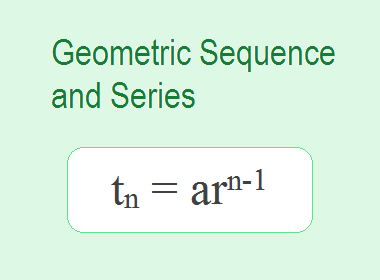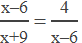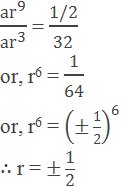# Geometric Sequence and SeriesConsider the following sequences.

a. 2, 4, 8, 16, … … … …

b. 27, 9, 3, 1, … … … …

c. 16, -24, 36, -54, … … … …

In the first sequence, each term is double of its previous term. In the second sequence, each term is one-third of its previous term. Each term of the third sequence is -3/2 times its previous term. In each of the above sequence, the ratio of a term and its previous term is equal or constant. Such a sequence is known as a Geometric Sequence or Geometric Progression.

## Definition

A sequence is said to be a geometric sequence or geometric progression if the ratio between any term and its previous term is equal or constant throughout the whole sequence. It is denoted by GP.

The constant ratio obtained by dividing a term by its previous term is called common ratio of the sequence. In above example 2, 4, 8, 16, … … the common ratio is 4/2 = 8/4 = 16/8 = 2. Similarly, the common ratio of 27, 9, 3, 1, … … is 1/3 and that of 16, -24, 36, -54, … …  is -3/2. The common ratio of a geometric sequence is denoted by r.

A series corresponding to any geometric sequence is known as the geometric series associated with the given geometric sequence. Hence, 2 + 4 + 8 + 16 + … … … is a geometric series associated with the geometric sequence 2, 4, 8, 16, … … … .********************

********************

## General Term of a GP

If a be the first term and r be the common ratio of a geometric progression, then the terms of the progression are: a, ar, ar2, ar3, … … …

If t1, t2, t3, t4, … … …, tn be the first, second, third, fourth, … … …, nth terms of a GP respectively then,

t1 = a = ar1-1

t2 = ar = ar2-1

t3 = ar2 = ar3-1

t4 = ar3 = ar4-1

… … … … … … …

tn = arn-1

Thus, if the first term and the common ratio of a GP are known, we can find any term.

## Worked Out Examples

Example1: If the first term and the common ratio of a GP are 1/8 and -2 respectively, find the seventh term of the GP.

Solution:

Here,

First term (a) = 1/8

Common ratio (r) = -2

Seventh term (t7) = ?

By using formula, we have,

tn = arn-1

or, t7 = 1/8 × (-2)7-1

or, t7 = 1/8 × (-2)6

or, t7 = 1/8 × 64

or, t7 = 8

Seventh term t7 = 8.

Example 2: For a geometric series 1/18 + 1/6 + 1/2 + … … … + 81/2

a. Find the number of terms.

b. Find the 9th term of the series.

Solution:

a.

Here,

The first term (a) = 1/18

Common ratio (r) = (1/6)/(1/18) = 3

Last term (tn) = 81/2

Number of terms (n) = ?

By using formula,

tn =arn-1

or, 81/2 = 1/18 × 3n-1

or, 81 × 9 = 3n-1

or, 3n-1 = 36

or, n – 1 = 6

or, n = 7

Number of terms n = 7

b.

9th term (t9) = ?

By using formula,

tn = arn-1

or, t9 = 1/18 × 39-1

or, t9 = 1/18 × 38

or, t9 = 1/18 × 6561

or, t9 = 729/2

9th term t9 = 729/2

Example 3: Is 1458 a term of the series 2 + 6 + 18 + 54 + … … … ?

Solution:

Here,

The series is 2 + 6 + 18 + 54 + … … …

The first term (a) = 2

Common ratio (r) = 6/2 = 3

Suppose, tn = 1458

or, arn-1 = 1458

or, 2 × 3n-1 = 1458

or, 3n-1 = 1458/2

or, 3n-1 = 729

or, 3n-1 = 36

or, n – 1 = 6

or, n = 7

1458 is the 7th term of the given series.

Example 4: Find the value of x for which x + 9, x – 6, 4 are the three terms of a geometric progression and calculate the fourth term of geometric progression in each case.

Solution:

The given three terms in GP are x + 9, x – 6, 4.

From the definition of GP,or, (x – 6)2 = 4(x + 9)

or, x2 – 12x + 36 = 4x + 36

or, x2 – 16x = 0

or, x(x – 16) = 0

x = 0 or 16

Case I,

When x = 0, the given numbers are 9 , -6, 4

First term (a) = 9

Common ratio (r) = -2/3

Fourth term (t4) = ?

We have, tn = arn-1

or, t4 = 9 × (-2/3)4-1

or, t4 = 9 × (-2/3)3

or, t4 = 9 × -8/27

t4 = -8/3

Case II,

When x = 16, the given numbers are 25, 10, 4

First term (a) = 25

Common ratio (r) = 2/5

Fourth term (t4) = ?

We have, tn = arn-1

or, t4 = 25 × (2/5)4-1

or, t4 = 25 × (2/5)3

or, t4 = 25 × (8/125)

t4 = 8/5

Example 5: If the fourth and tenth terms are 32 and 1/2 respectively, find the GP. Also find its 15th term.

Solution:

Let a be the first term and r be the common ratio of the GP.

Fourth term (t4) = 32

Tenth term (t10) = ½

We have,

tn = arn-1

or, t4 = ar4-1

or, ar3 = 32 … … … (i)

and, t10 = ar10-1

or, ar9 = 1/2 … … … (ii)

Dividing equation (ii) by (i),Case I,

When r = ½

ar3 = 32

or, a × 1/8 = 32

or, a = 256

The given GP is 256, 128, 64, … … …

And,

t15 = ar15-1

or, t15 = 256 × (1/2)14

or, t15 = 256 × 1/16384

t15 = 1/64

Case II,

When r = -1/2

ar3 = 32

or, a × (-1/2)3 = 32

or, a × -1/8 = 32

or, a = -256

The given GP is -256, 128, -64, … … …

And,

t15 = ar15-1

or, t15 = -256 × (-1/2)14

or, t15 = -256 × 1/16384

t15 = -1/64

Example 6: In a geometric series, 7th term is 16 times of the third term and the fifth term is 1/16, find the second term.

Solution:

Here,

t7 = 16 × t3

or, ar6 = 16 × ar2

or, ar6/ar2 = 16

or, r4 = 16 = 24

r = 2.

Now,

t5 = 1/16

or, ar4 = 1/16

or, ar × r3 = 1/16

or, t2 × 23 = 1/16

or, t2 × 8 = 1/16

or, t2 = 1/128

Second term t2 = 1/128

Example 7: The product of three numbers in GP is 1000. If we add 6 to its second number and 7 to its third number, the resulting three numbers form an AP. Find the numbers in GP.

Solution:

Let the numbers in GP be a/r, a and ar.

Then, a/r × a × ar = 1000

or, a3 = 1000

a = 10

Hence the numbers are 10/r, 10 and 10r.

The new numbers are 10/r, 10 + 6, and 10r + 7 and these numbers are in AP.

16 – 10/r = (10r + 7) – 16

or, 16r – 10 = 10r2 – 9r

or, 10r2 – 25r + 10 = 0

or, 2r2 – 5r + 2 = 0

or, 2r2 – 4r – r + 2 = 0

or, 2r(r – 2) – 1(r – 2) = 0

or, (r – 2)(2r – 1) = 0

r = 2 or 1/2

Case I,

When r = 2, the numbers are 10/2, 10, 10×2 i.e. 5, 10, 20

Case II,

When r = 1/2, the numbers are 10/(1/2), 10, 10×1/2 i.e. 20, 10, 5

Hence, the numbers are 5, 10, 20 or 20, 10, 5.